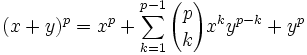## Thursday, May 25, 2006

### Cyclotomic Integers: g(α)p ≡ g(αp) (mod p)

In today's blog, I continue to review results from Harold Edward's Fermat's Last Theorem: A Genetic Introduction to Algebraic Number Theory. I am continuing down the same chapter that I started in an earlier blog. If you would like to start at the beginning of cyclotomic integer properties, start here.

Lemma 1: if p is a prime, then (x + y)p ≡ xp + yp

Proof:

(1) Using the Binomial Theorem, we know that:(2) Now, this means that each of the coefficients is equal to:(3) But since p is a prime, p divides all coefficients.

For all values where k is between 1 and p-1, p, as a prime, will not be divisible by either k! or by (p-k)!.

(4) This means that p will divides (x + y)p - xp - yp.

QED

Lemma 2: xp ≡ x (mod p)

Proof:

(1) xp - x = x(xp-1 - 1)

(2) if gcd(x,p)=1, then by Fermat's Little Theorem, xp-1 ≡ 1 (mod p) so p divides xp-1-1.

(3) if p divides x, then of course p divides x(xp-1-1)

QED

Lemma 3: g(α) ≡ g(αp) mod p

Proof:

(1) Let g(α) = a0 + a1α + ... + αλ-1αλ-1.

(2) Then g(α)p = (a0 + [a1α + ... + αλ-1αλ-1])p

(3) Now using Lemma 1 above, we can break #2 out in the following ways:

(a0 + [a1α + ... + αλ-1αλ-1])p ≡ (a0)p + (a1α + [... + αλ-1αλ-1])p (mod p).

(4) We could keep breaking out each element until we get:

(a0 + [a1α + ... + αλ-1αλ-1])p ≡ (a0)p + (a1α)p + ... + (aλ-1αλ-1)p (mod p)

(5) Now for each coefficient ai, using Lemma 2 above, we have:

aip ≡ ai (mod p)

So this gives us:

(a0)p + (a1α)p + ... + (aλ-1αλ-1)p ≡ a0 + a1αp + ... + aλ-1λ-1)p (mod p)

(6) And we are done since this shows that:

g(α)p ≡ g(αp) (mod p)

QED